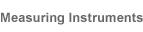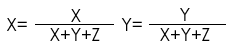## Language of Light

## 2.Concepts

### 2.4 COLORIMETRY

#### 2.4.4.1 TRISTIMULUS COLORIMETRY

##### 2.4.4.1.1 CIE 1931 Yxy CHROMATICITY CHART

The tristimulus values XYZ are useful for defining a colour, but the results are not easily visualised. Because of this, CIE defined a colour space in 1931 for graphing colour into two dimensions independent of lightness; this is the Yxy colour space, in which Y is the lightness and x and y are the chromaticity coordinates calculated from the tristimulus value XYZ.The x and y chromaticity coordinates are calculated from the XYZ tristimulus values according to the following formulae:The principal drawback of the 1931 system is that equal distances on the chart do not represent equal perceived colour differences because of non-linearities in the human eye.